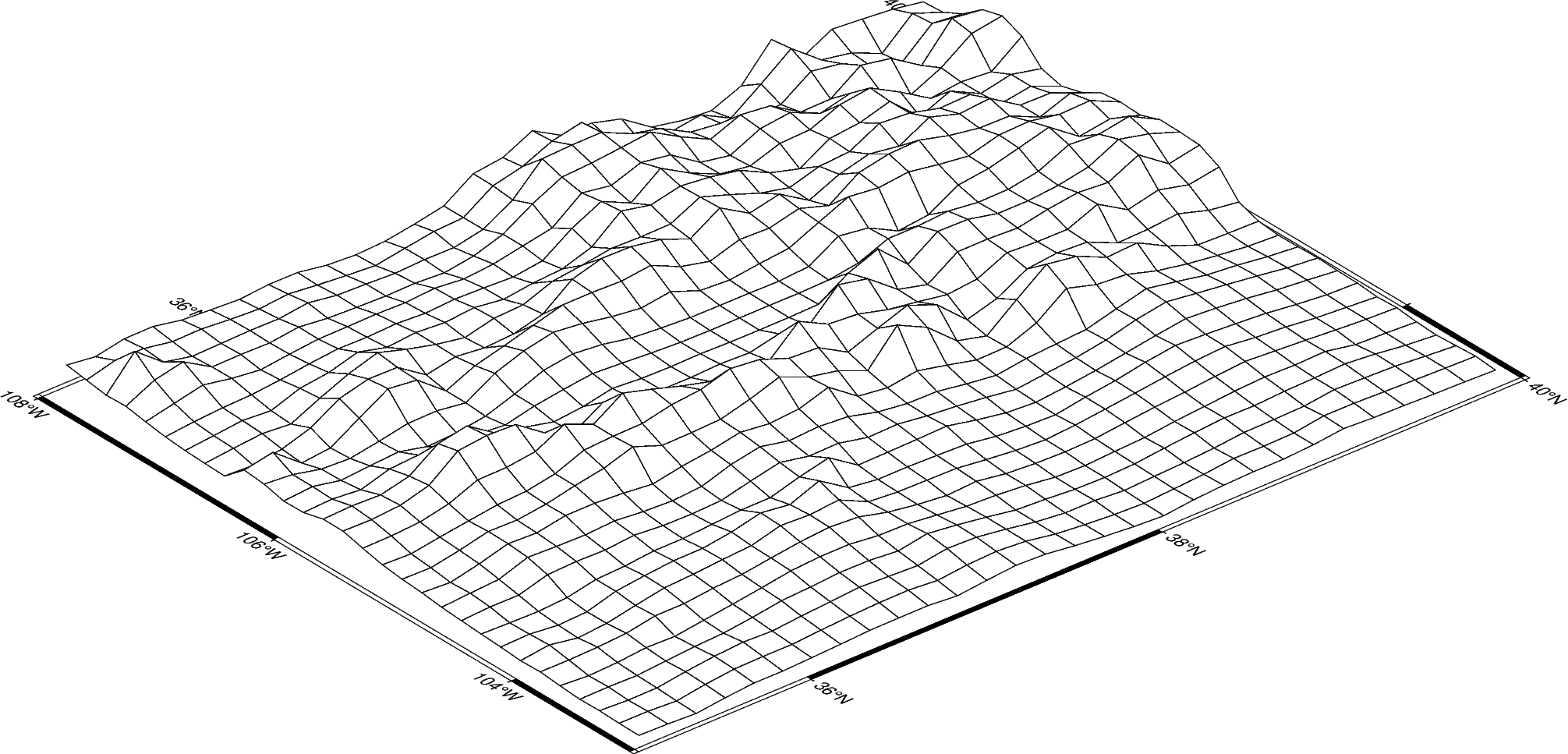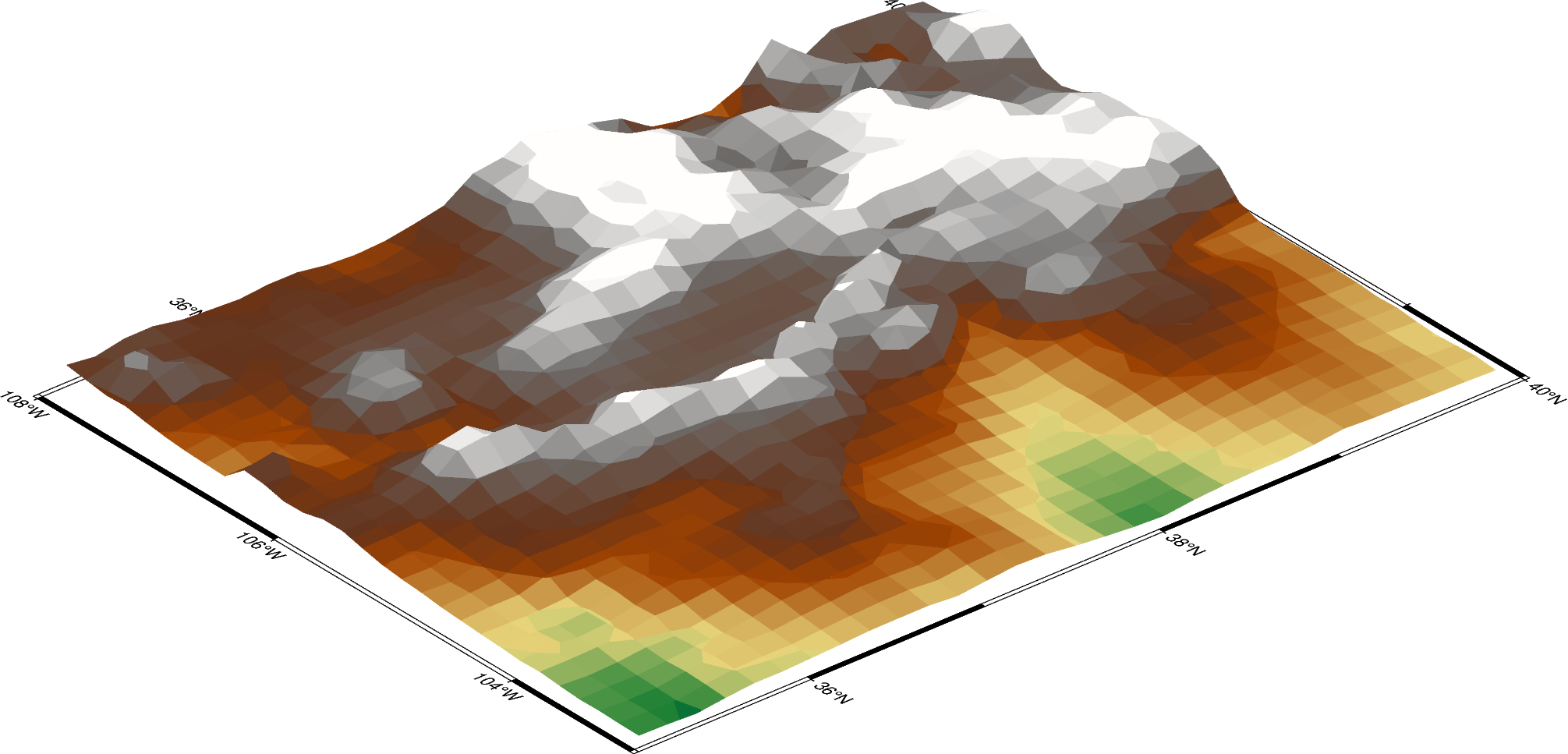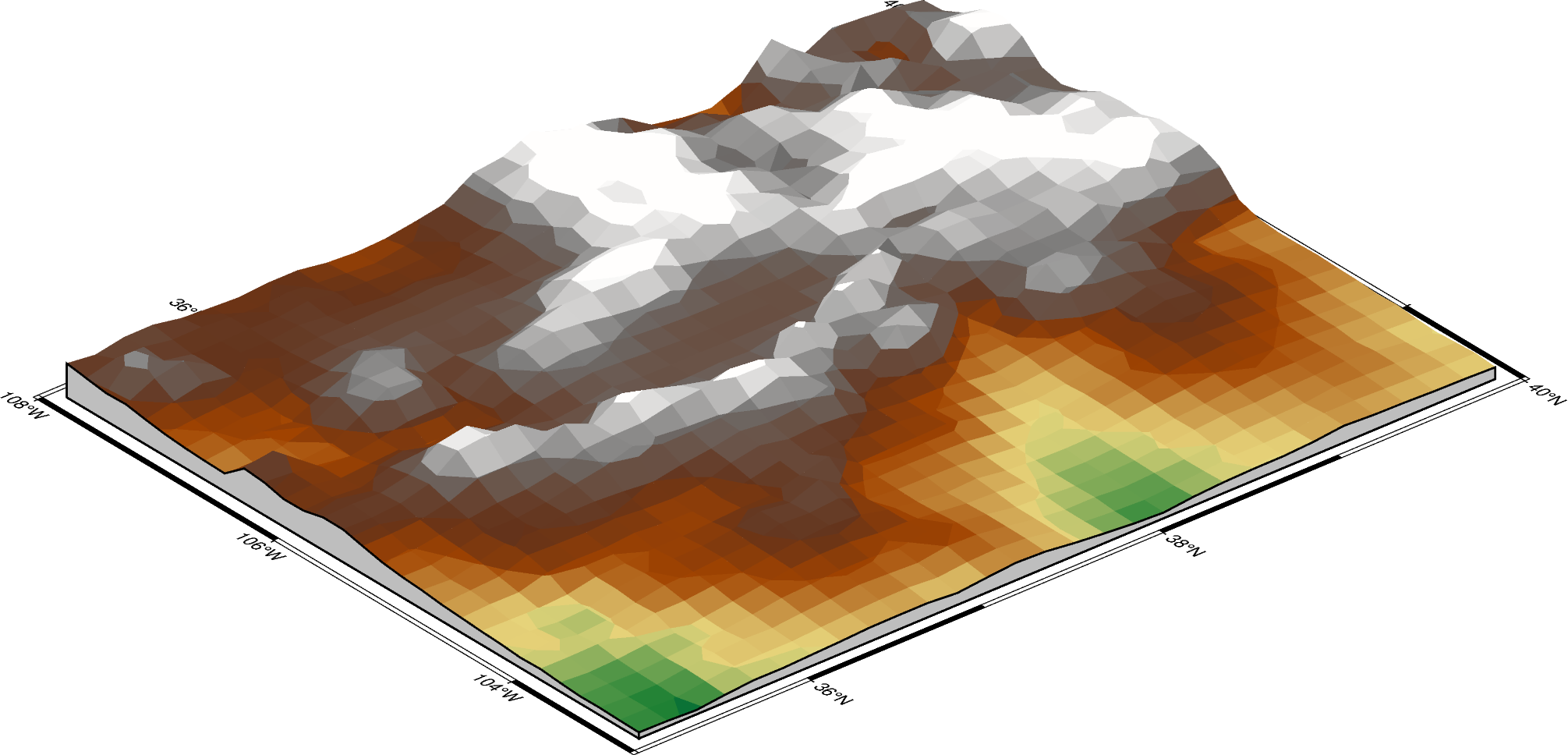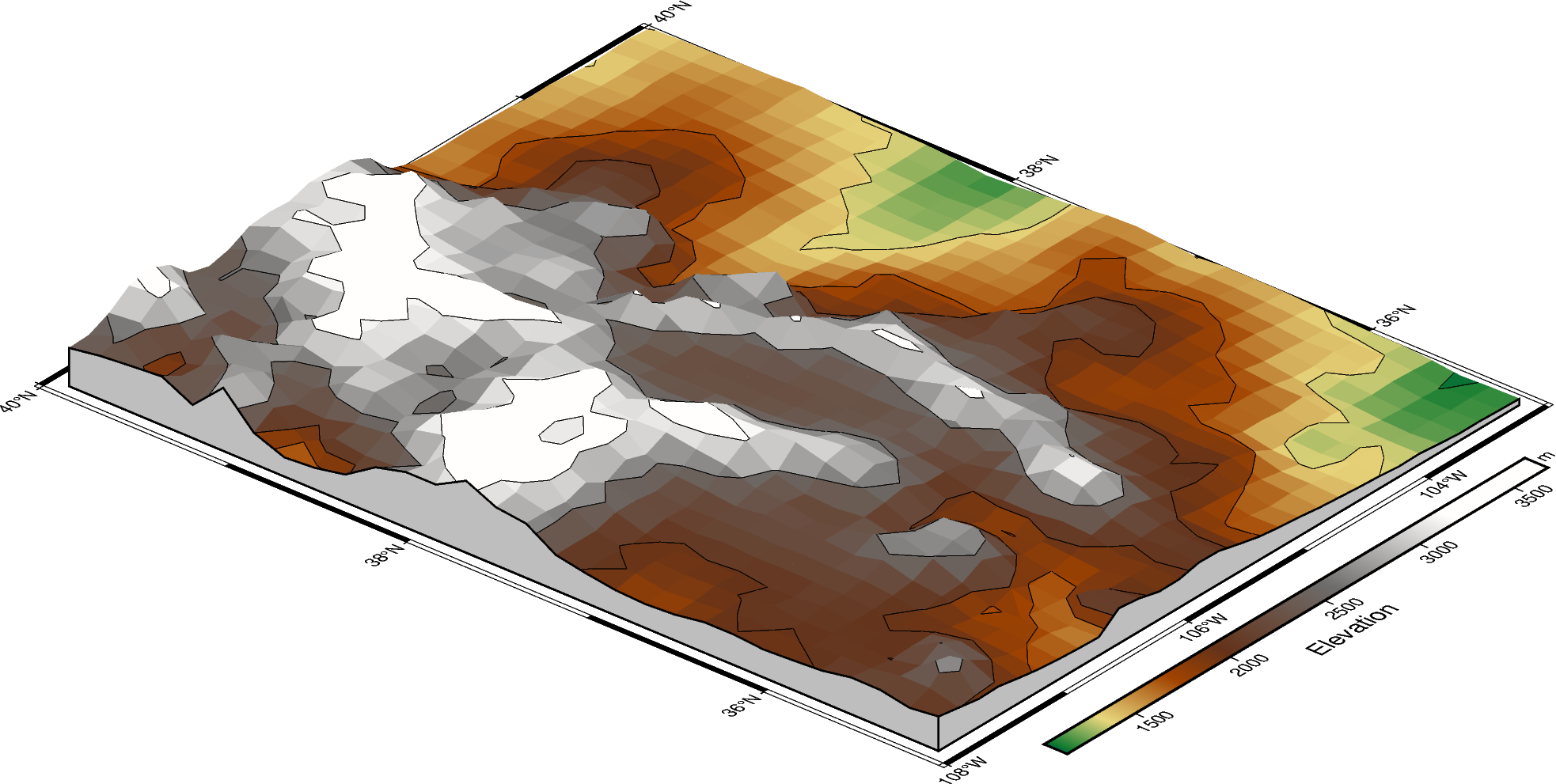Creating a 3D perspective image

Create 3-D perspective image or surface mesh from a grid using pygmt.Figure.grdview.

import pygmt

# Load sample earth relief data
grid = pygmt.datasets.load_earth_relief(resolution="10m", region=[-108, -103, 35, 40])

The pygmt.Figure.grdview method takes the grid input. The perspective parameter changes the azimuth and elevation of the viewpoint; the default is [180, 90], which is looking directly down on the figure and north is “up”. The zsize parameter sets how tall the three-dimensional portion appears.

The default grid surface type is mesh plot.

fig = pygmt.Figure()
fig.grdview(
grid=grid,
# Sets the view azimuth as 130 degrees, and the view elevation as 30
# degrees
perspective=[130, 30],
# Sets the x- and y-axis labels, and annotates the west, south, and east
# axes
frame=["xa", "ya", "WSnE"],
# Sets a Mercator projection on a 15-centimeter figure
projection="M15c",
# Sets the height of the three-dimensional relief at 1.5 centimeters
zsize="1.5c",
)
fig.show()Out:

<IPython.core.display.Image object>

The grid surface type can be set with the surftype parameter. The default CPT is turbo and can be customized with the cmap parameter.

fig = pygmt.Figure()
fig.grdview(
grid=grid,
perspective=[130, 30],
frame=["xa", "yaf", "WSnE"],
projection="M15c",
zsize="1.5c",
# Set the surftype to "surface"
surftype="s",
# Set the CPT to "geo"
cmap="geo",
)
fig.show()Out:

<IPython.core.display.Image object>

The plane parameter sets the elevation and color of a plane that provides a fill below the surface relief.

fig = pygmt.Figure()
fig.grdview(
grid=grid,
perspective=[130, 30],
frame=["xa", "yaf", "WSnE"],
projection="M15c",
zsize="1.5c",
surftype="s",
cmap="geo",
# Set the plane elevation to 1,000 meters and make the fill "gray"
plane="1000+ggray",
)
fig.show()Out:

<IPython.core.display.Image object>

The perspective azimuth can be changed to set the direction that is “up” in the figure. The contourpen parameter sets the pen used to draw contour lines on the surface. pygmt.Figure.colorbar can be used to add a color bar to the figure. The cmap parameter does not need to be passed again. To keep the color bar’s alignment similar to the figure, use True as the perspective parameter.

fig = pygmt.Figure()
fig.grdview(
grid=grid,
# Set the azimuth to -130 (230) degrees and the elevation to 30 degrees
perspective=[-130, 30],
frame=["xaf", "yaf", "WSnE"],
projection="M15c",
zsize="1.5c",
surftype="s",
cmap="geo",
plane="1000+ggrey",
# Set the contour pen thickness to "0.1p"
contourpen="0.1p",
)
fig.colorbar(perspective=True, frame=["a500", "x+lElevation", "y+lm"])
fig.show()Out:

<IPython.core.display.Image object>

Total running time of the script: ( 0 minutes 4.861 seconds)

Gallery generated by Sphinx-Gallery